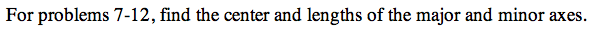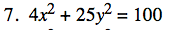### Home > PC > Chapter 13 > Lesson 13.1.2 > Problem13E-7

13E-7.Refer to Example 3 in this section to assist you with this problem.

Divide everything by the constant, 100 to make the equation = 1.

$\frac{4\textit{x}^2}{100}+\frac{25\textit{y}^2}{100}=\frac{100}{100}$